﻿ 海底管道单、双腐蚀剩余强度影响研究
 舰船科学技术2022, Vol. 44Issue (14): 107-112    DOI: 10.3404/j.issn.1672-7649.2022.14.023PDF

Research on influence of single and double corrosion on residual strength of submarine pipeline
WANG Hong, HU Wei-li
School of Ship and Ocean Engineering, Jiangsu University of Science and Technology, Zhenjiang, 212003
Abstract: In order to study the influence of single and double corrosion on the residual strength of submarine pipeline, this paper will take the buried submarine pipeline with corrosion defects as an example, and use Abaqus finite element software to model and analyze it. In Abaqus software, after setting the basic parameters of pipeline and corrosion defects, the finite element simulation analysis of five groups of different single corrosion and double corrosion under normal operation condition and limit operation condition is carried out respectively, and then the residual strength of submarine pipeline is obtained according to the results of limit operation condition. Finally, the influence of single corrosion and double corrosion on the residual strength of submarine pipeline is analyzed by using the calculation results of single corrosion and double corrosion .The difference of the residual strength between the two kinds of corrosion can be compared, and some suggestions are put forward.
Key words: corrosion     Abaqus     finite element     normal operating condition     limit operating condition
0 引　言

1 工程概况

1.1 基本模型参数表 1 管道材料属性 Tab.1 Pipeline material properties
1.2 材料的本构关系

 $\varepsilon = \left\{\begin{split} &{\varepsilon }_{e}+{\varepsilon }_{p}=\frac{\sigma }{{E}_{0}}\left[1+\frac{8}{15}\times {\left(\frac{\sigma }{450}\right)}^{14}\right]，\quad\quad\quad\;\;\sigma \leqslant {\sigma }_{0.2}，\\ & \frac{\sigma -450}{16\;535.43} + 0.005\;47\times {\left(\frac{\sigma -450}{45.43}\right)}^{28} + 0.002\;8 ，\sigma > {\sigma }_{{0.2}}。\end{split} \right.$ (1)

2 数值模拟 2.1 有限元模型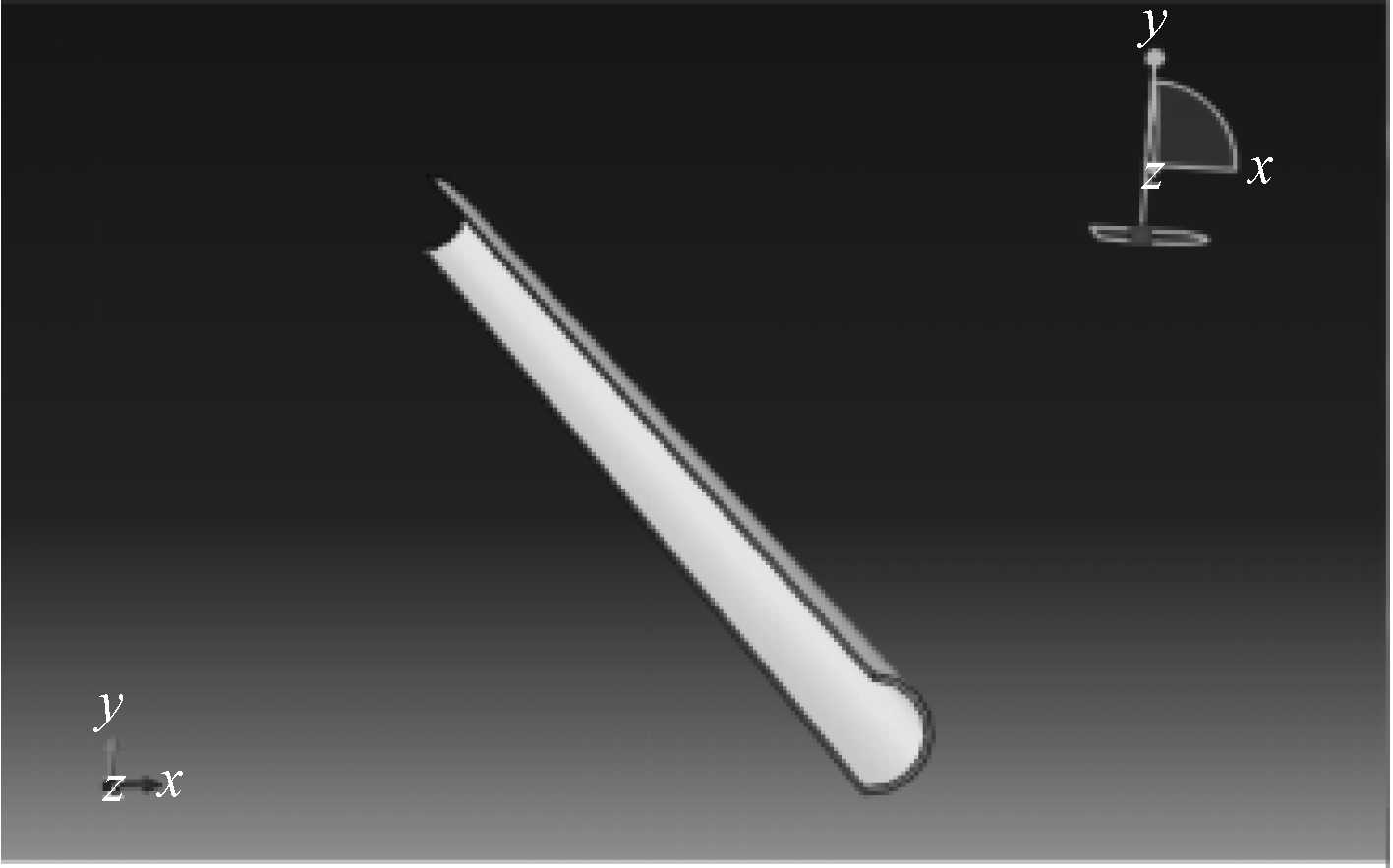图 1 1 m海底管道的1/2结构模型示意图 Fig. 1 Schematic diagram of 1/2 structure model of 1 m subsea pipeline
2.2 建模步骤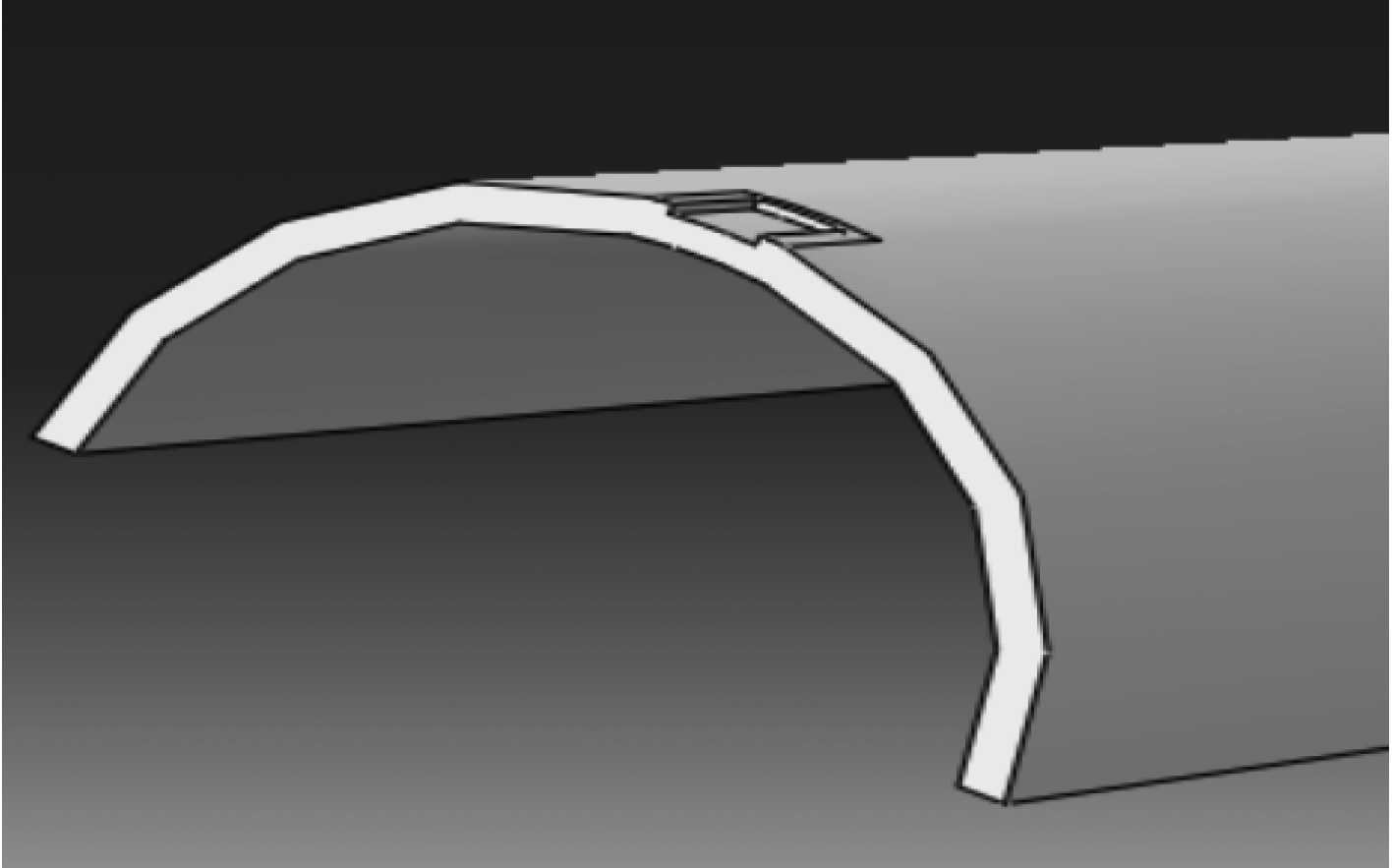图 2 1/2腐蚀模型示意图 Fig. 2 1/2 corrosion pit model diagram

 ${\sigma _i} = \frac{{2{\sigma _{{b}}}\left( {L \times {{t}} - \displaystyle\sum\limits_i {{d_i}{l_i}} } \right)}}{{L \times \left( {D - 2{{t}}} \right)}} 。$ (2)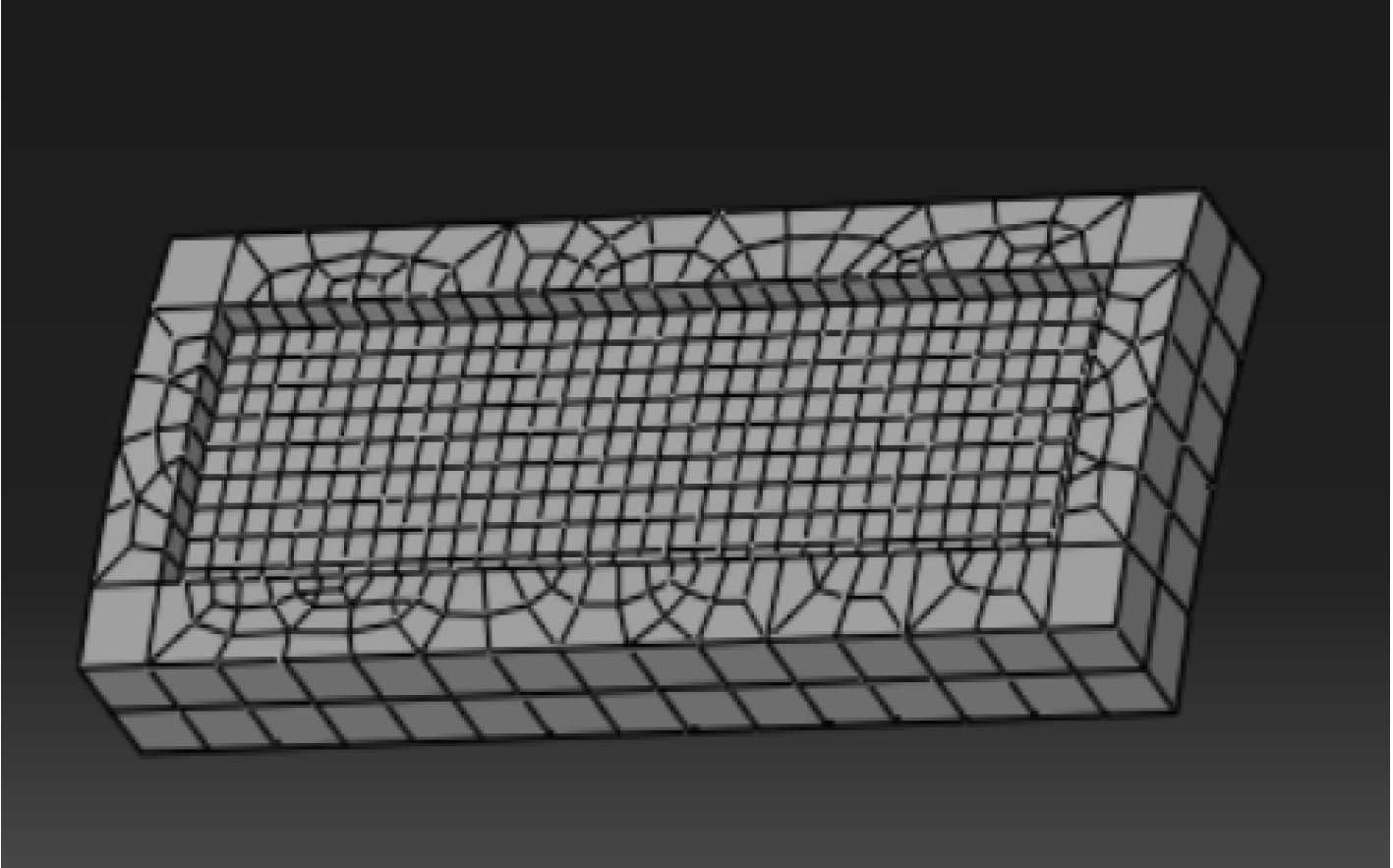图 3 腐蚀区域加密示意图 Fig. 3 Schematic diagram of corrosion area encryption

3 结果分析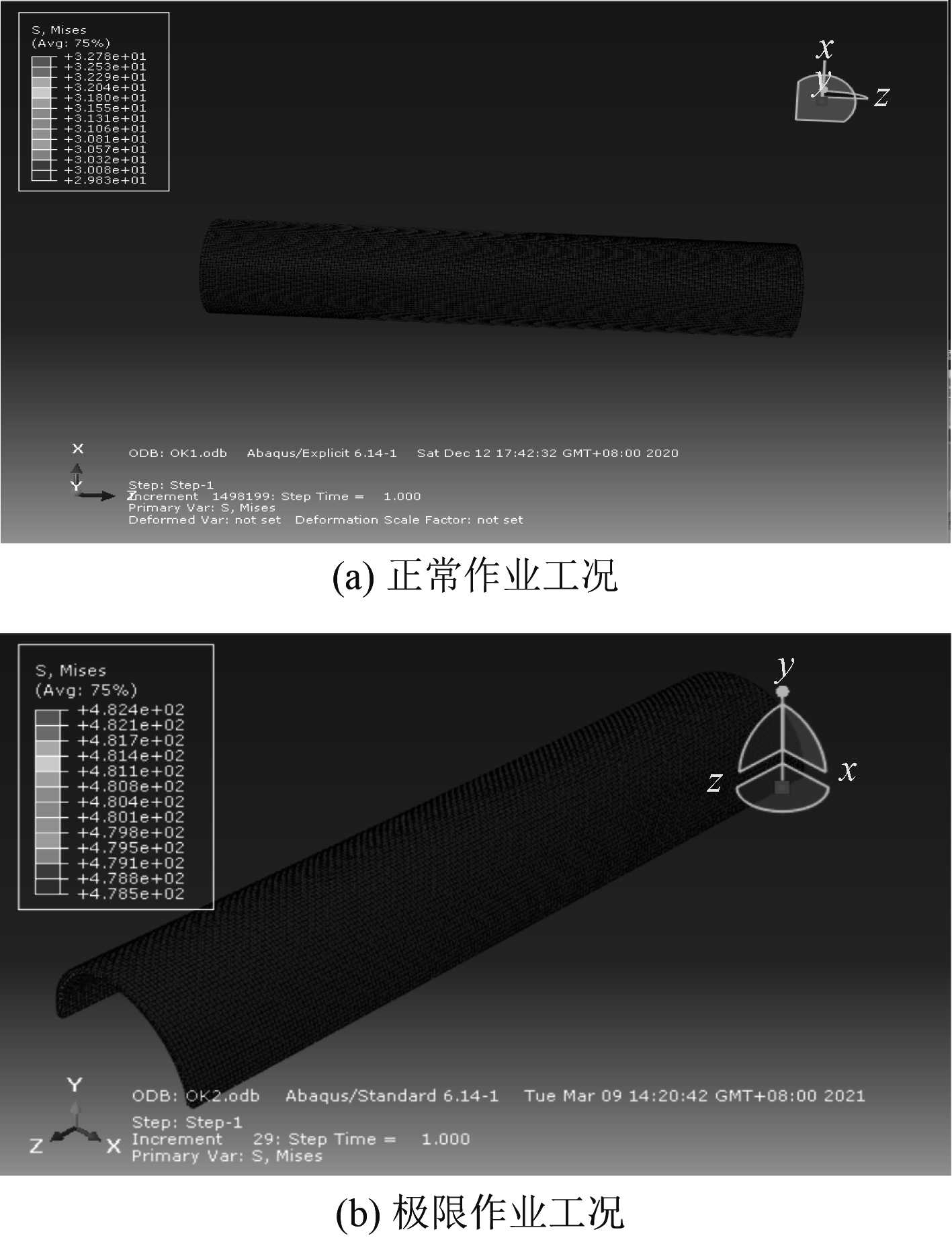图 4 无缺陷管道应力云图示意图 Fig. 4 Stress cloud atlas of pipeline without defects
3.1 单腐蚀计算结果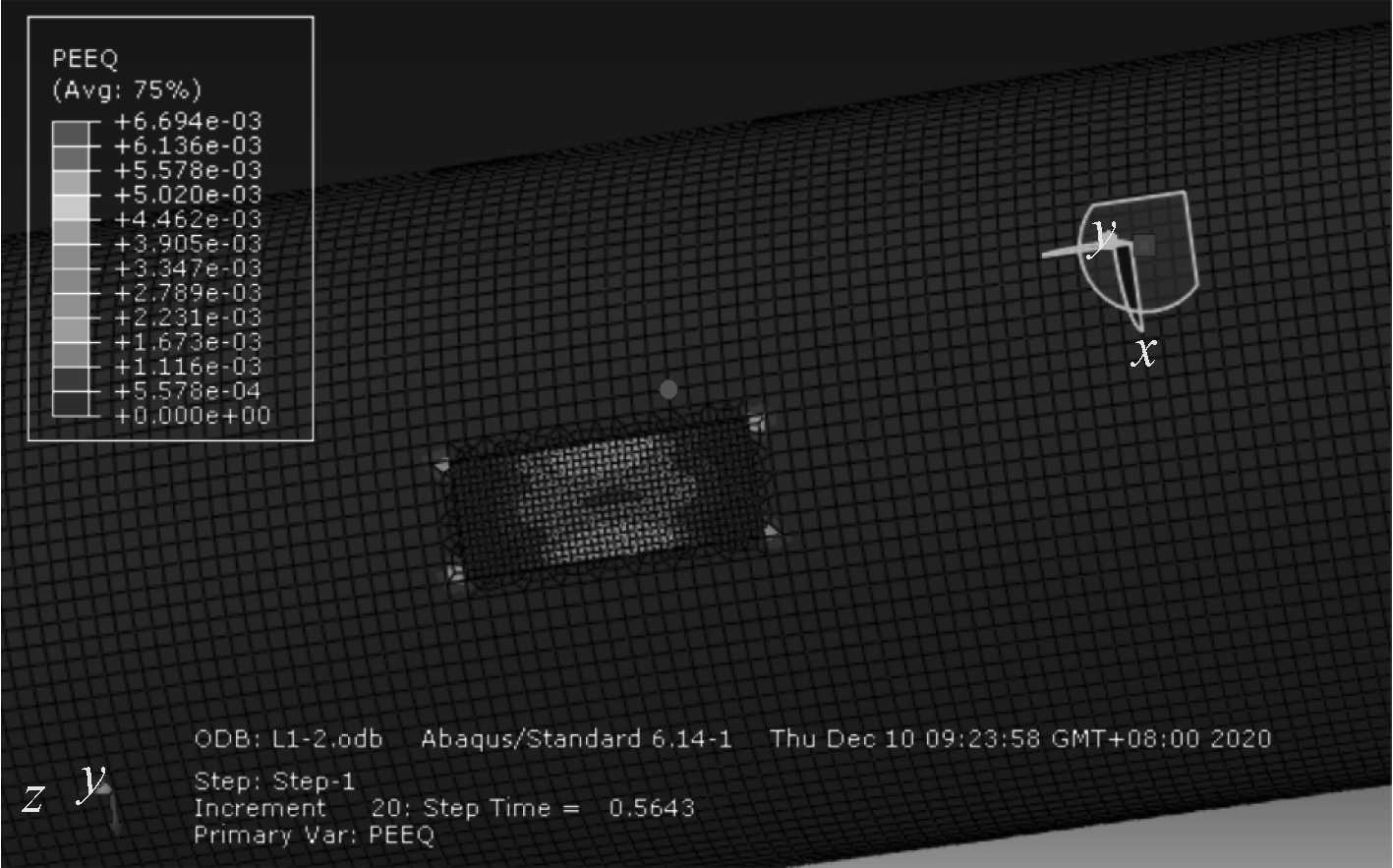图 6 L1塑性变形示意图 Fig. 6 Plastic deformation diagram of L1
 ${\sigma _{{\text{li}}}} = T \times {\sigma _i}。$ (3)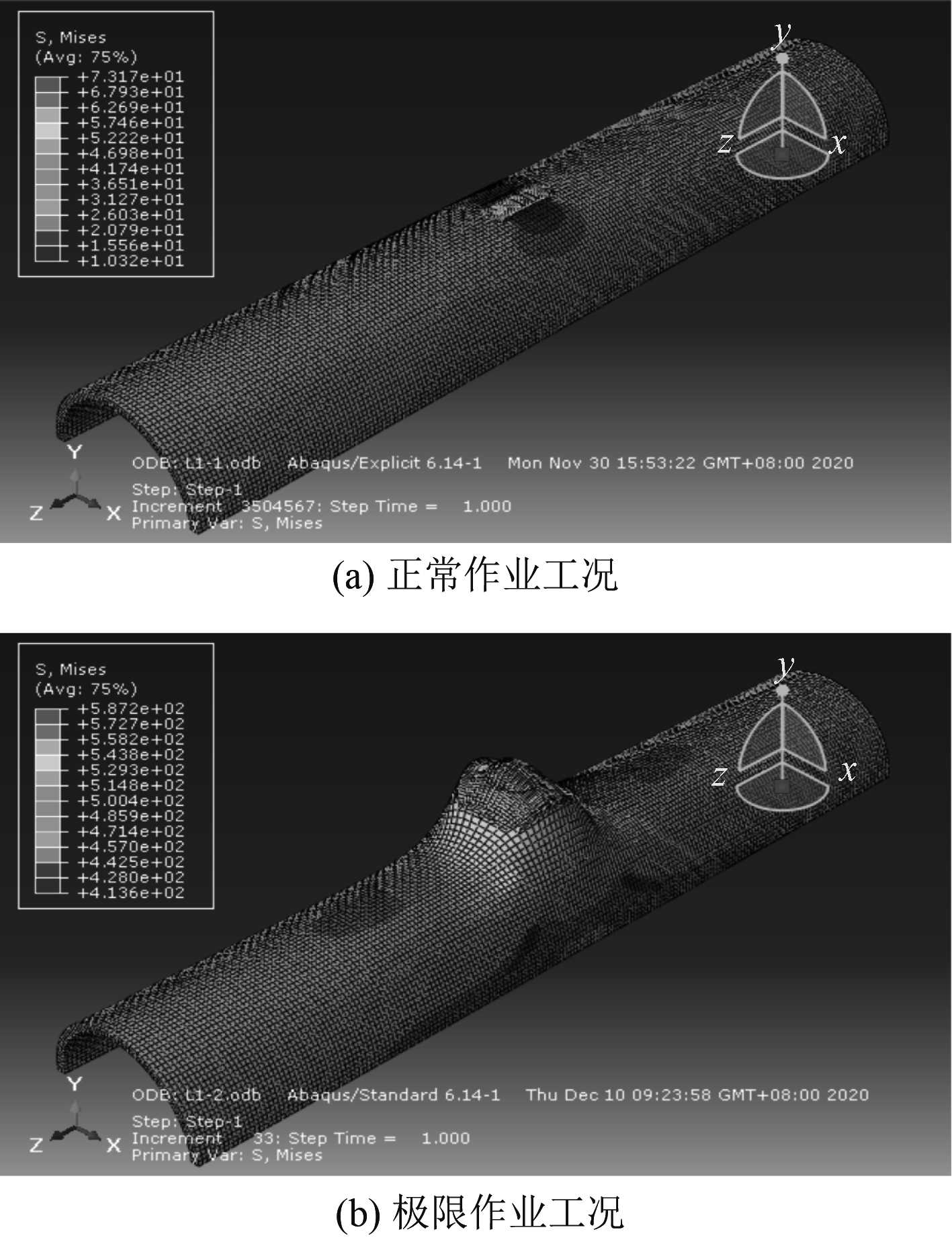图 5 L1应力云图示意图 Fig. 5 Stress cloud atlas of L1表 2 单腐蚀在2种工况下的计算结果 Tab.2 Calculation results of single corrosion pit under two working conditions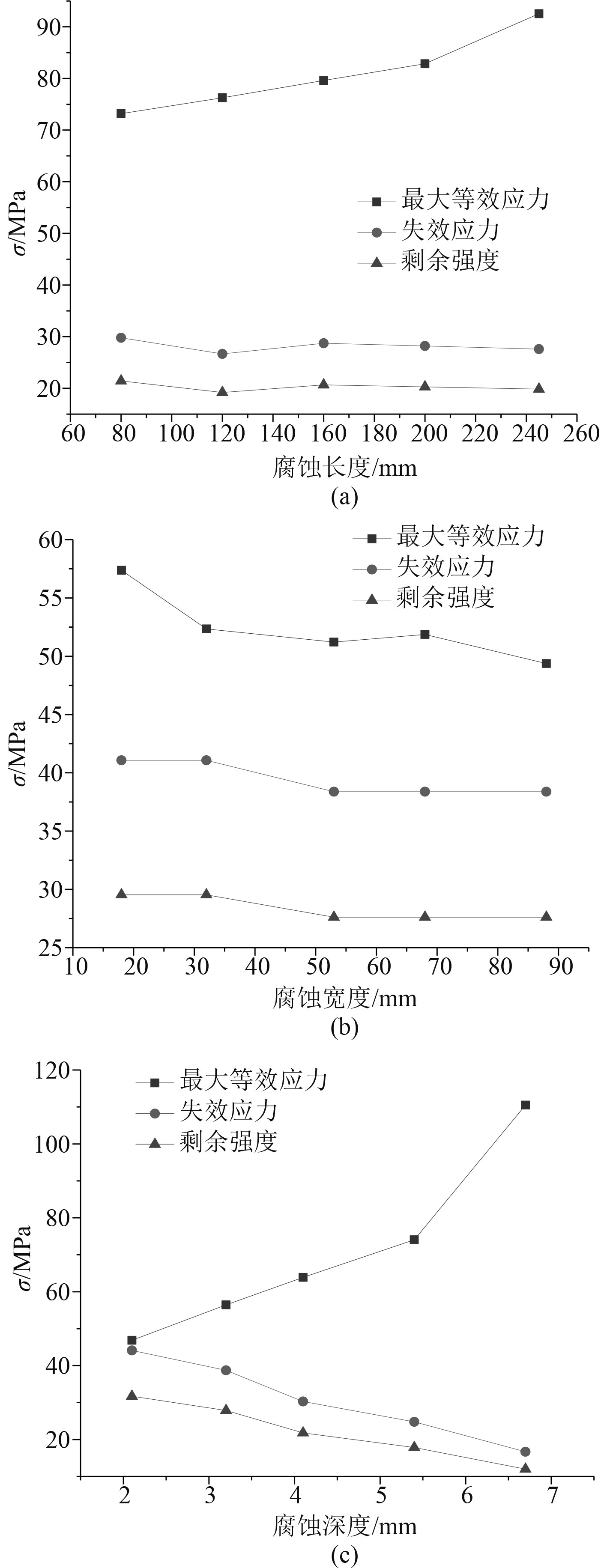图 7 单腐蚀计算结果折线图 Fig. 7 Calculation result of single corrosion
3.2 单腐蚀结果分析

1）不同长度的单腐蚀

2）不同宽度的单腐蚀

3）不同深度的单腐蚀

3.3 双腐蚀计算结果表 3 双腐蚀在2种工况下的计算结果 Tab.3 Calculation results of double corrosion pit under two working conditions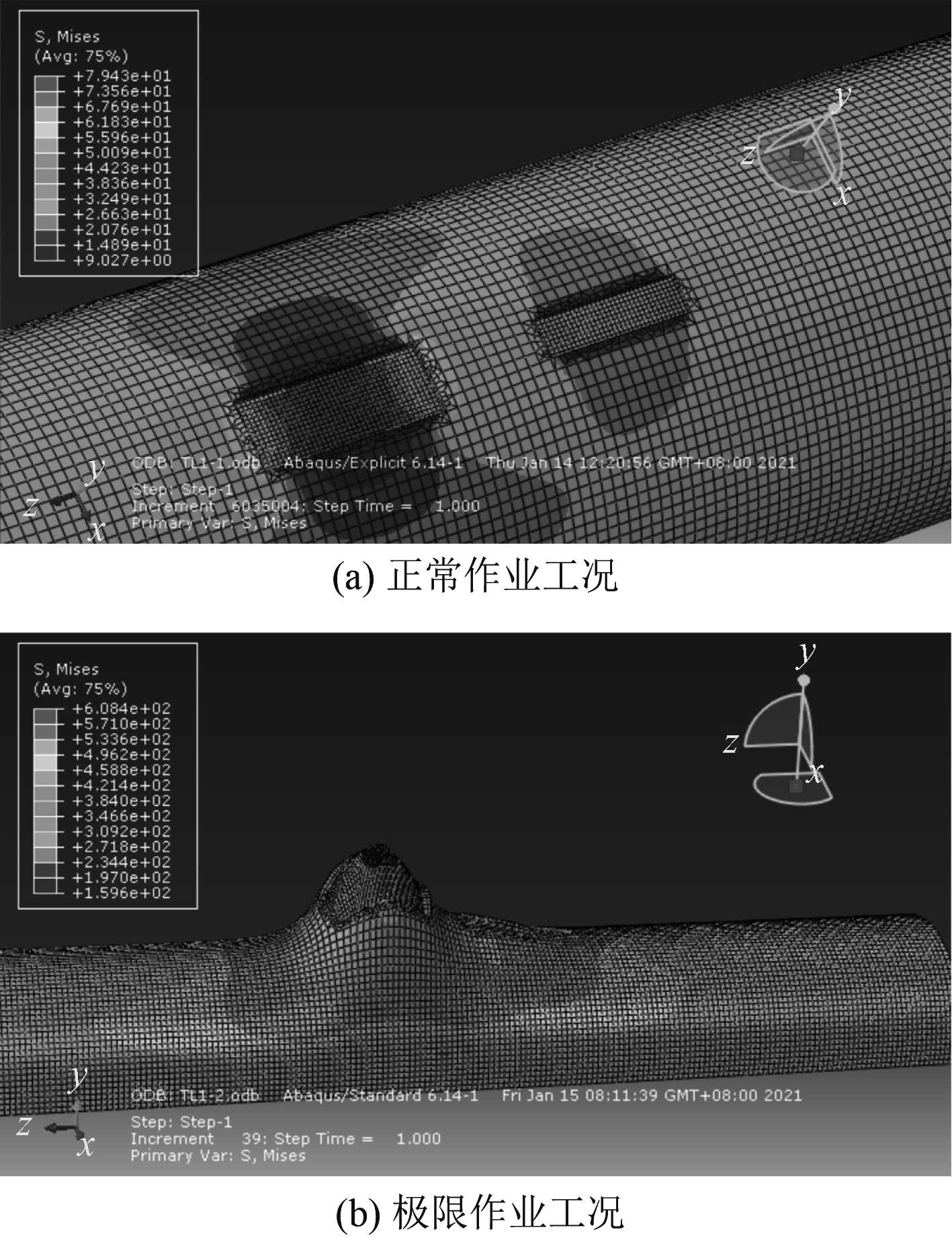图 8 TL1应力云图示意图 Fig. 8 Stress cloud atlas of TL1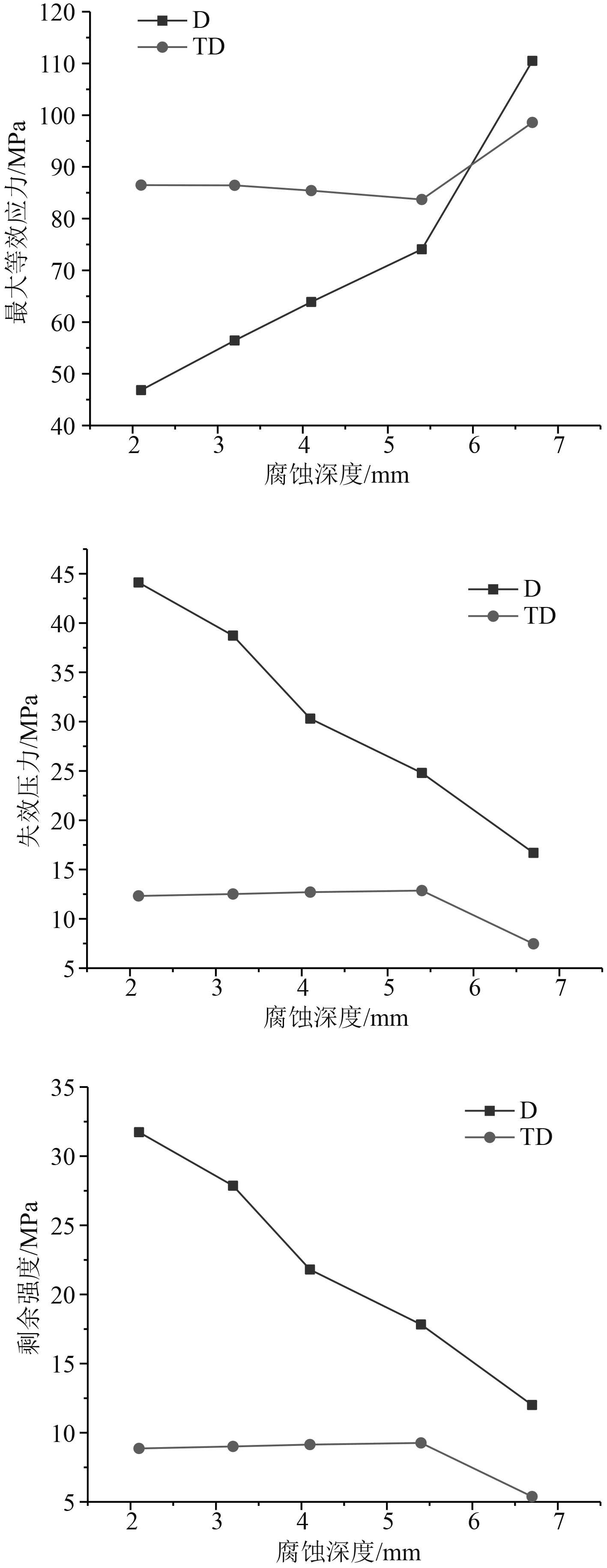图 9 单、双腐蚀对比折线图 Fig. 9 Single and double corrosion Comparison line chart
3.4 单、双腐蚀结果分析

1）不同长度的单腐蚀与不同长度、宽度的双腐蚀

2）不同宽度的单腐蚀与不同宽度、深度的双腐蚀

3）不同深度的单腐蚀与不同深度、长度的双腐蚀

4 结　语

1）单腐蚀的最大等效应力即使出现增大的现象，但是均小于管道的屈服强度与极限抗拉伸强度，这说明管道仍可以服役。单腐蚀的失效压力与剩余强度明显下降仅出现在腐蚀的深度增大，说明腐蚀深度越深越容易出现应力集中现象即腐蚀深度对海底管道的剩余强度影响较大。但是表2中剩余强度值均大于管道的最大运行压力，这意味目前管道有充足的强度可以继续使用。

2）双腐蚀的最大等效应力均大于单腐蚀的等效应力，但是如果管道腐蚀的长度超过220 mm或者深度超过6 mm时会出现双腐蚀的等效应力不大于单腐蚀的等效应力。从失效压力与剩余强度的角度看，双腐蚀均小于单腐蚀，但是可以发现如果双腐蚀的尺寸相差较大时，双腐蚀的剩余强度明显低于单腐蚀的剩余强度，尤其是双腐蚀中某个腐蚀坑的深度超过6.7 mm时甚至可能出现失效的情况。

  王超众, 谢维纶, 崔鹏, 等. 海底管道完整性管理技术及其应用[J]. 中国石油和化工标准与质量, 2013, 33(12): 186-187. DOI:10.3969/j.issn.1673-4076.2013.12.174  段安琪. 腐蚀海底管道安全评价方法的研究[D]. 青岛: 中国石油大学, 2017.  马钢, 白瑞. 海底腐蚀管道剩余强度评估方法对比[J]. 焊管, 2018, 41(9): 25-29.  黄坤, 李沅桦, 孔令圳. 基于参考应力法的海底腐蚀管道剩余强度评价[J]. 中国安全生产科学技术, 2017, 13(7): 163-167.  卢盛灿, 张军, 龚翔, 等. 腐蚀海底管道剩余强度分析[J]. 船海工程, 2016, 45(1): 142-146.  吴龙飞. 基于有限元分析的海底管道剩余强度预测方法研究[D]. 杭州: 浙江工业大学, 2019.  王煜博. BZ34-1至友谊号海底管道的安全评价技术研究[D]. 成都: 西南石油大学, 2014.  徐震. X65管线钢的本构关系及失效判据研究[J]. 石油工程建设, 2014, 40(3): 23-27. DOI:10.3969/j.issn.1001-2206.2014.03.006